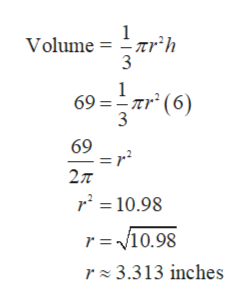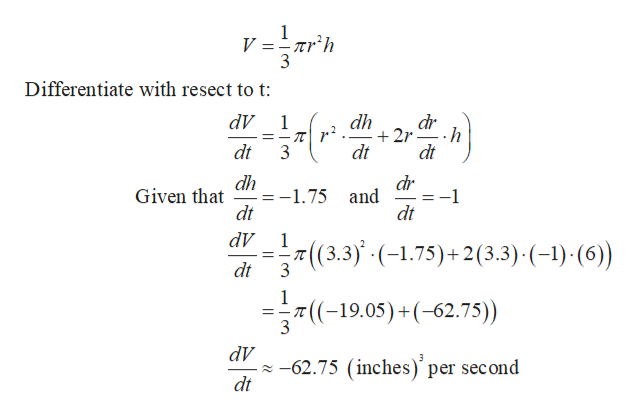# You and your friends are designing and constructing a cone-shaped funnel. Whatever you use this funnel for is up to you. You decide that the height of the funnel will be 6 inches.(a) What does the radius of the base of the funnel need to be if you want the volume of it to be 69 inches cubed? (V = 1/3 πr 2h, where r is the radius of the circular base, and h is the height.)(b) You finally construct it, with the correct height and radius, and you pour the liquid into it to test it out. Again, the choice of liquid and the reason you are doing this is up to you. In measuring the effectiveness of the funnel, you determine that as the liquid flows out of the bottom, the radius of the liquid as it’s in the funnel is decreasing at a rate of 1 inch per second, and the height is decreasing at a rate of 1.75 inches per second. Find the rate at which the volume of the liquid (in inches cubed per second) is decreasing.(c) How many seconds will it take for the funnel to be empty at this rate?

Question
4 views

You and your friends are designing and constructing a cone-shaped funnel. Whatever you use this funnel for is up to you. You decide that the height of the funnel will be 6 inches.

(a) What does the radius of the base of the funnel need to be if you want the volume of it to be 69 inches cubed? (V = 1/3 πr 2h, where r is the radius of the circular base, and h is the height.)

(b) You finally construct it, with the correct height and radius, and you pour the liquid into it to test it out. Again, the choice of liquid and the reason you are doing this is up to you. In measuring the effectiveness of the funnel, you determine that as the liquid flows out of the bottom, the radius of the liquid as it’s in the funnel is decreasing at a rate of 1 inch per second, and the height is decreasing at a rate of 1.75 inches per second. Find the rate at which the volume of the liquid (in inches cubed per second) is decreasing.

(c) How many seconds will it take for the funnel to be empty at this rate?

check_circle

Step 1

Part (a):

Let r is the radius and h is height of the funnel and it is given that height of the funnel is 6 inches:

Volume of the cone shaped funnel:help_outlineImage TranscriptioncloseVolume = rr’h 3 69 = ar" (6) ar` (6) 3 69 = r? r' = 10.98 r =V10.98 r z 3.313 inches fullscreen
Step 2

Part (b):

It is given that the height is decreasing at a rate of 1.75 inches per second. The radius of the liquid is decreasing at a ...help_outlineImage TranscriptioncloseDifferentiate with resect to t: dr + 2r dt dV dh 1 T| r? . ..h dt dt dr dh :-1.75 and dt Given that dt dV T7 (3.3) ·(-1.75)+2(3.3)·(-1)·(6) dt 3 = 7((-19.05)+(-62.75)) 3 dV z -62.75 (inches)' per second dt fullscreen

### Want to see the full answer?

See Solution

#### Want to see this answer and more?

Solutions are written by subject experts who are available 24/7. Questions are typically answered within 1 hour.*

See Solution
*Response times may vary by subject and question.
Tagged in

### Calculus